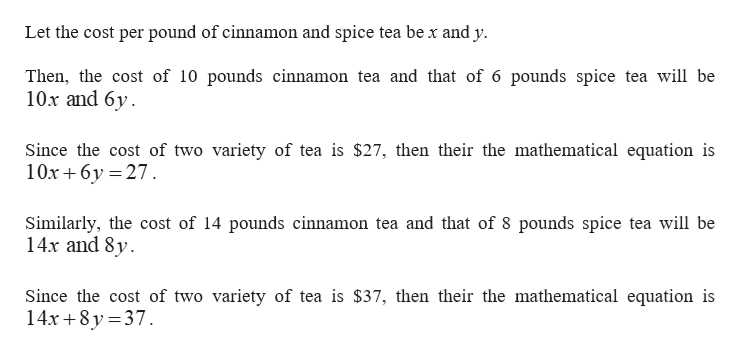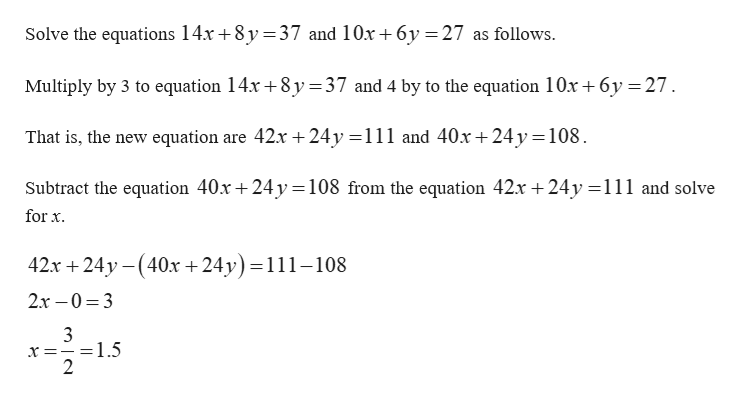# A merchant mixed 10 lb of a cinnamon tea with 6 lb of spice tea. The 16-pound mixture cost \$27. A second mixture included 14 lb of the cinnamon tea and 8 lb of the spice tea. The 22-pound mixture cost \$37. Find the cost per pound of the cinnamon tea and of the spice tea.

Question
14 views

A merchant mixed 10 lb of a cinnamon tea with 6 lb of spice tea. The 16-pound mixture cost \$27. A second mixture included 14 lb of the cinnamon tea and 8 lb of the spice tea. The 22-pound mixture cost \$37. Find the cost per pound of the cinnamon tea and of the spice tea.

check_circle

Step 1help_outlineImage TranscriptioncloseLet the cost per pound of cinnamon and spice tea be x and y Then, the cost of 10 pounds cinnamon tea and that of 6 pounds spice tea will be 10x and 6y Since the cost of two variety of tea is \$27, then their the mathematical equation is 10x6y 27 Similarly, the cost of 14 pounds cinnamon tea and that of 8 pounds spice tea will be 14x and 8y Since the cost of two variety of tea is \$37, then their the mathematical equation is 14x+8y 37 fullscreen
Step 2help_outlineImage TranscriptioncloseSolve the equations 14x +8y 37 and 10x 6y 27 as follows - Multiply by 3 to equation 14x 8y =37 and 4 by to the equation 10x 6y 27 That is, the new equation are 42x 24y -111 and 40x +24y 108 Subtract the equation 40x +24y108 from the equation 42x 24y =111 and solve forx 42х + 24у - (40х + 24у) %3D111-108 2х —0%3 3 3 =1.5 2 X fullscreen

### Want to see the full answer?

See Solution

#### Want to see this answer and more?

Solutions are written by subject experts who are available 24/7. Questions are typically answered within 1 hour.*

See Solution
*Response times may vary by subject and question.
Tagged in

### Other